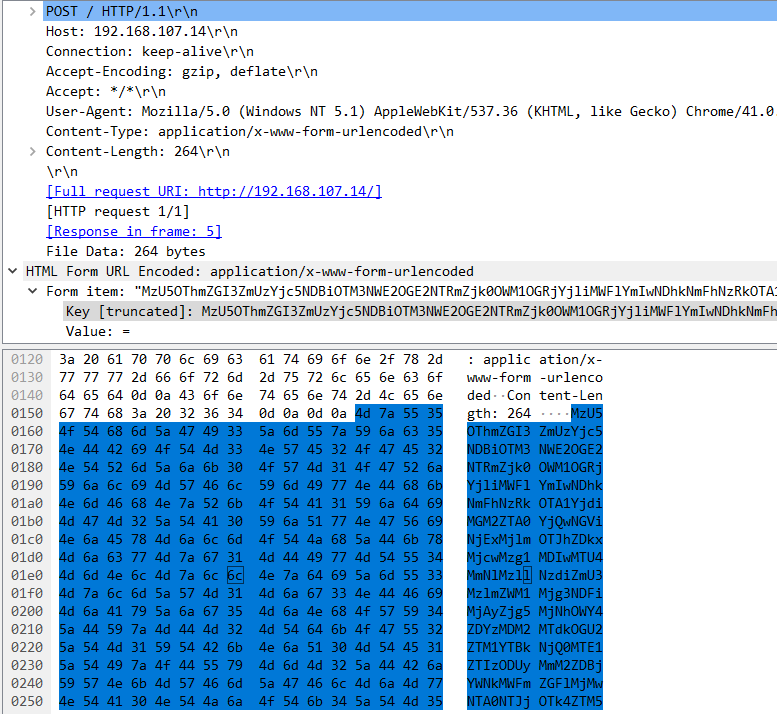Article List

## Results

I have a lot of upcoming tests and personal stuff, and I'm losing my focus on many other things. Anyways, I somehow spared a very bit of time in TMCTF this weekend.

I didn't solve a lot of problems due to lack of time.. I should try better and faster next time.This writeup consists of analysis-offense 200pt, reverse-binary 100pt, forensic-crypto1 200pt and forensic-crypto2 200pt.

## Analysis-Offensive 200pt

I just modified my callgrind solver (gist) to solve this challenge.

python
``````\$ cat oracle.py
#!/usr/bin/python -u
#-*- coding:utf-8 -*-

# Let's exploit easy and quick!
# 1) apt install valgrind
# 2) use callgrind to find instruction count

flag = 'TMCTF{'
n = 0

import os
import sys

charset = "abcdefghijklmnopqrstuvwxyzABCDEFGHIJKLMNOPQRSTUVWXYZ0123456789{}"
while True:
n += 1
total_call_count = {}
for i in charset:
cmd = "valgrind --tool=callgrind --dump-instr=yes --callgrind-out-file=temp/call_count ./oracle '" + flag + i + "A'  2>&1"
# print(cmd)

call_count = res.split("Collected : ").split()
call_count = int(call_count)
# total_call_count { 'call_count': [occured_count, occured_by], ... }
if not total_call_count.get(call_count):
total_call_count[call_count] = [1, [i]]
else:
total_call_count[call_count] += 1
total_call_count[call_count].append(i)
print(n, i, call_count)
## get lowest/highest idx,
idx_call_count = total_call_count.keys()
print(idx_call_count)
idx_call_count.sort()
highest_count_idx = idx_call_count[-1]
lowest_count_idx = idx_call_count
# get highest idx
flag_char = total_call_count[highest_count_idx]
flag += flag_char
print(n, total_call_count, highest_count_idx, flag)

``````

## Reverse-Binary 100pt

We first find base64-encoded data from the pcap file.Then, reverse the pyinstaller binary and modify the script to solve the challenge.

python
``````import struct, os, time, threading, urllib, requests, ctypes, base64
from Cryptodome.Cipher import AES, ARC4
from Cryptodome.Hash import SHA

infile = 'flag'
encfile = 'orig.CRYPTED'
keyfile = 'keyfile'
sz = 1024
bs = 16

def decrypt_request():
pcap_req = ("35998fdb7fe3b7940b9375a68a654ff949c58dcb9b1aebb048d6aa74d905b7b0c6e04b404eb61129f92" +
_hash_chksum = pcap_req[:40]
_hash_content = pcap_req[40:]
dec = ARC4.new(_hash_chksum.decode('hex'))
return dec.decrypt(_hash_content.decode('hex'))

def generate_keyfile():
# n = hex(ord(id) + bs)
n = hex(ord('d1'.decode('hex')) + 16)
iv = "95bc0ed56ab0e730b64cce91c9fe9390".decode('hex')

key = ''.join((chr(ord(x) ^ int(n, 16)) for x in key))
iv = ''.join((chr(ord(y) ^ int(n, 16)) for y in iv))
keyfile = open("keyfile", "wb")
keyfile.write(key + iv)
keyfile.close()
print(n, iv, key)
return True

def decrypt():
global keyfile
key = ''
iv = ''
if not os.path.exists(encfile):
exit(0)
while True:
time.sleep(10)
if os.path.exists(keyfile):
keyin = open(keyfile, 'rb')
if len(key) != 0 and len(iv) != 0:
aes = AES.new(key, AES.MODE_CBC, iv)
fin = open(encfile, 'r')
fout = open(infile, 'w')
fin.seek(2, 0)
while True:
n = len(data)
if n == 0:
break
decrypted = aes.decrypt(data)
n = len(decrypted)
if fsz > n:
fout.write(decrypted)
else:
fout.write(decrypted[:fsz])
fsz -= n

fin.close()
os.remove(encfile)
break

print(decrypt_request())
generate_keyfile()
decrypt()

# ----Trend Micro CTF 2018. Flag for this challenge is: TMCTF{MJB1200}
``````

## Forensics-crypto1 200pt

Decompiling pyinstaller shows the sourcecode.

python
``````\$ cat OceanOfSockets.py

...
def request():
try:
connection = httplib.HTTPConnection(sys.argv, sys.argv)
connection.request('GET', '/tmctf.html')
resTMCF = connection.getresponse()
headers = {'User-Agent': 'Mozilla Firefox, Edge/12',
'Content-type': 'text/html',
else:
sys.exit(0)
except:
pass
...
``````

There doesn't seem to be much information except the suspicious cookie.

While thinking about the flag format (which is `TMCTF{}`), I realized it should be a simple addition algorithm used on Cookie.

python
``````>>> [chr((ord(i) + 47)) for i in '%|r%uL5bbA0F?5bC0E9b0_4b2?N']
['T', '\xab', '\xa1', 'T', '\xa4', '{', 'd', '\x91', '\x91', 'p', '_', 'u', 'n', 'd', '\x91', 'r', '_', 't', 'h', '\x91', '_', '\x8e', 'c', '\x91', 'a', 'n', '}']
``````

Now it sounds like some of characters are not displayed properly. I decided to mod a byte to leak remaining ambiguous bytes.

python
``````>>> [chr((ord(i) + 47) % 0x5e) for i in '%|r%uL5bbA0F?5bC0E9b0_4b2?N']
['T', 'M', 'C', 'T', 'F', '\x1d', '\x06', '3', '3', '\x12', '\x01', '\x17', '\x10', '\x06', '3', '\x14', '\x01', '\x16', '\n', '3', '\x01', '0', '\x05', '3', '\x03', '\x10', '\x1f']

``````

Merging above results will print the flag

flag: `TMCTF{d33p_und3r_th3_0c3an}`

## Forensics-crypto2 200pt

Dump HTTP upload requests and you will get mausoleum.exe.From there, decompile the exe file (omg so many pyinstaller binary)I was somehow unable to decrypt the python file (I changed some of bits in headers, still it didn't work)

so I decided to remove useless letters from the notepad and get the flag. Guess what? I successfully submitted it on the first guess.

`TMCTF{the_s3cr3t_i\$_unE@rth3d}`